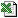Business Functions Library for Excel

Note: In order to use the spreadsheet examples, you need to download Business Functions
RateConvert (FromDatesInt, IntSimple, FromDate, ToDate, [DayCountSource], [DayCountTarget], PrdsSource, PrdsTarget, [MarginOpt])
Generic Rate Convert
Converts an Interest Rate from one basis of compounding to another, taking into account possible irregular compounding periods.
###### Why we need this function

• We need this function because sometimes when you are compounding from one interest basis (e.g. quarterly, ACT/365) to another (e.g. semi-annual, 30/360), you can"t do it using the normal algebraic formulae. Often you actually can"t do it without working out the interest in detail for the exact period you are looking at. This function effectively works out the interest on two completely different compounding regimes, and gives the interest rate that makes the Target regime equivalent to the first.
• Furthermore, where you have a separate margin that is needed for compounding the balance but where you don"t want to compound interest on interest with this margin, the interest calculation has another step in it as the interest and interest on interest is compounded separately.
• RateConvert is the only generic way to accurately convert a wide range of interest rate types from one basis to another.

###### Methodology

• RateConvert is a highly generic function for converting any type of interest rate to any other.
• It uses proper DayCount and Period definition variables to define both the interest rate you are converting from (the Source) and that you are converting to (the Target)
• It returns the interest rate applicable to DayCountTarget, ŘPeriodsTargetŘ and ŘIntRatesSimpleŘ, between FromDate and ToDate.
• The optional MarginOpt variable is to determine how interest on interest is calculated. If you use MarginOpt, this margin is added to ŘIntRatesSimpleŘ to determine the interest, but the interest on interest is only compounded at ŘIntRatesSimpleŘ, without adding any extra margin.

###### Discussion

Converting from one interest rate basis to another can be extremely complex. For example, many functions in this family deal with the simple case where an interest rate is being converted from a simple interest rate applied at periodic intervals, to an annually in arrears AER (annual equivalent rate) interest rate. These other functions use annuity formulae of the kind (1+r/4)^m-1. These annuity formulae are generalisations and are not accurate when you have specific interest payment dates that may not be at exactly equal intervals, or when you have some of the more exotic daycount conventions.

This function is truly generic, but you need to understand what its doing.

• First, it calculates the interest between FromDate and ToDate on a example balance of \$1000 using ŘIntRatesSimpleŘ and the "Source" parameters. This is simple enough (it actually uses Con).
• If you use MarginOpt, the interest rate on the balance is (IntRate+MarginOpt) and the interest on interest is at IntRate.
• Then, it takes a guess at an interest rate for Target (ie the result), and calculates the interest as above.
• The two amounts of interest are different because the guessed rate is probably wrong. The correct interest rate is determined simply by saying: Result=IntRateGuess*IntSource/IntTarget.

###### Question

In what circumstances do you need to specify MarginOpt?RateConvert.xls